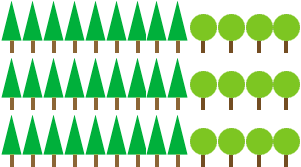I have this prolem and i don't understand.This is the problem. 1.A team of scientists found that in a forest there was four maple trees for every nine pine trees.How many maple were there if they counted 45 more pine than maples? a.65 b.13 c.45 d.36 Hi, There are 4 maple trees for each 9 pine trees so I see these trees in clusters of 9 + 4 = 13 trees.In each cluster of trees there are 4 maple trees and 9 pine trees, thus in each cluster there are 5 more pine trees than maple trees. In total you have 45 more pine trees than maple trees. How many clusters do you have? Cheers, Penny Go to Math Central